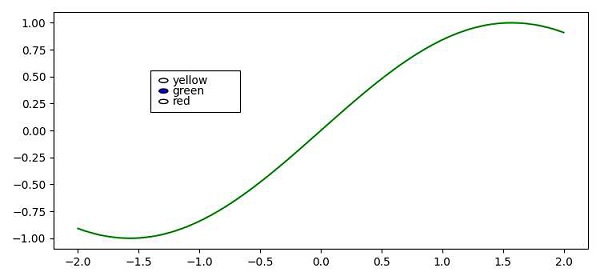# How to change the color of a line using radiobuttons in Matplotlib?

To change the color of a line using radiobutton we can take following steps −

• Create x and y data points using numpy.

• Adjust the figure size and padding between and around the subplots.

• Create a figure and a set of subplots using subplots() method.

• Plot curve with x and y data points using plot() method.

• Add an axes to the current figure and make it the current axes, using axes() method.

• Change the color of the curve with radion button using change_color() method, that can be passed in on_clicked() method.

• To display the figure use show() method.

## Example

import numpy as np
from matplotlib import pyplot as plt
plt.rcParams["figure.figsize"] = [7.50, 3.50]
plt.rcParams["figure.autolayout"] = True

x = np.linspace(-2, 2, 100)
y = np.sin(x)
fig, ax = plt.subplots()
curve, = ax.plot(x, y)
rb_axis = plt.axes([0.25, 0.60, 0.15, 0.15])

def change_color(c_label):
curve.set_color(c_label)
plt.draw()

plt.show()

## OutputNow, use the radiobuttons to change the color of the curve inside the plot.

Updated on: 09-Apr-2021

176 Views